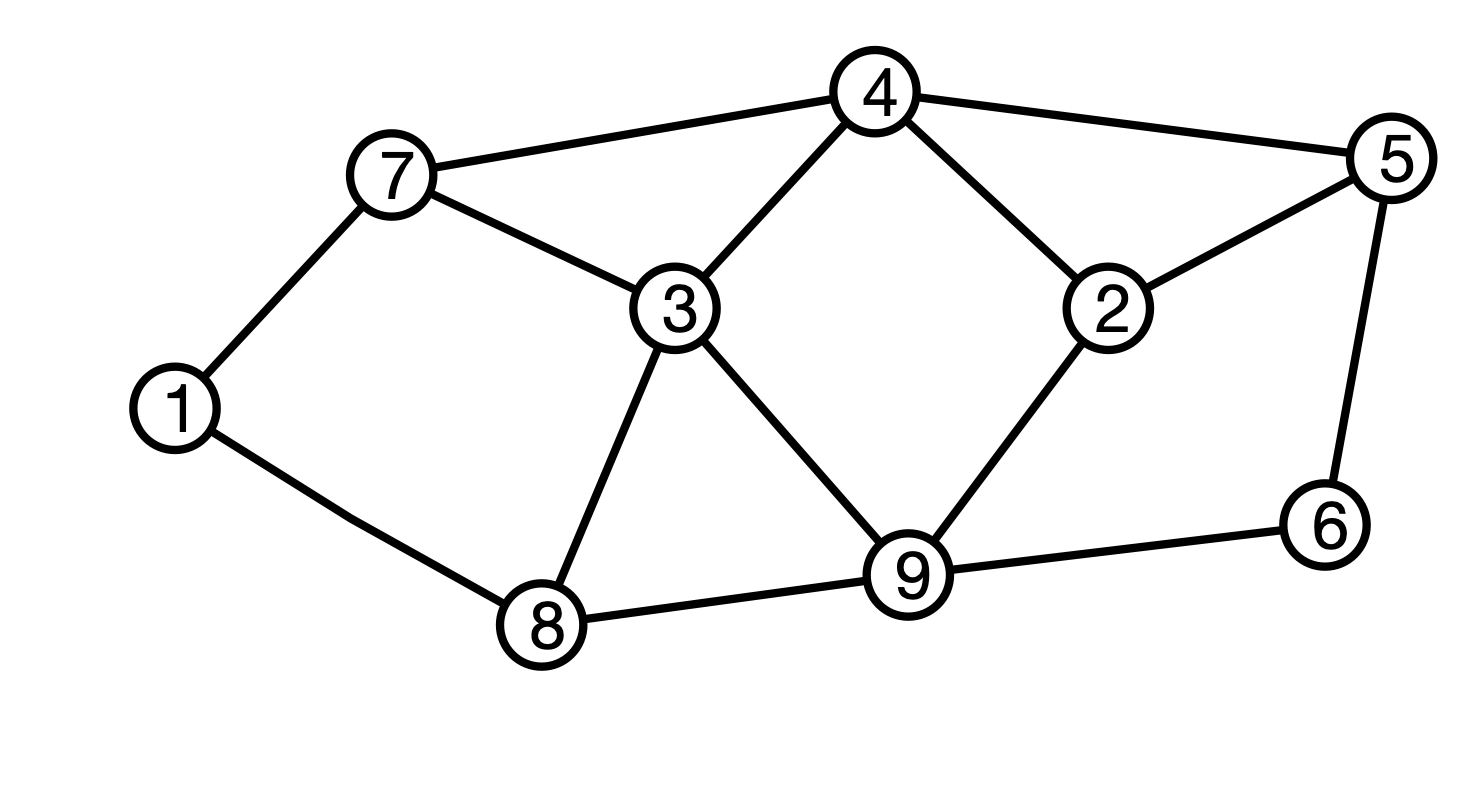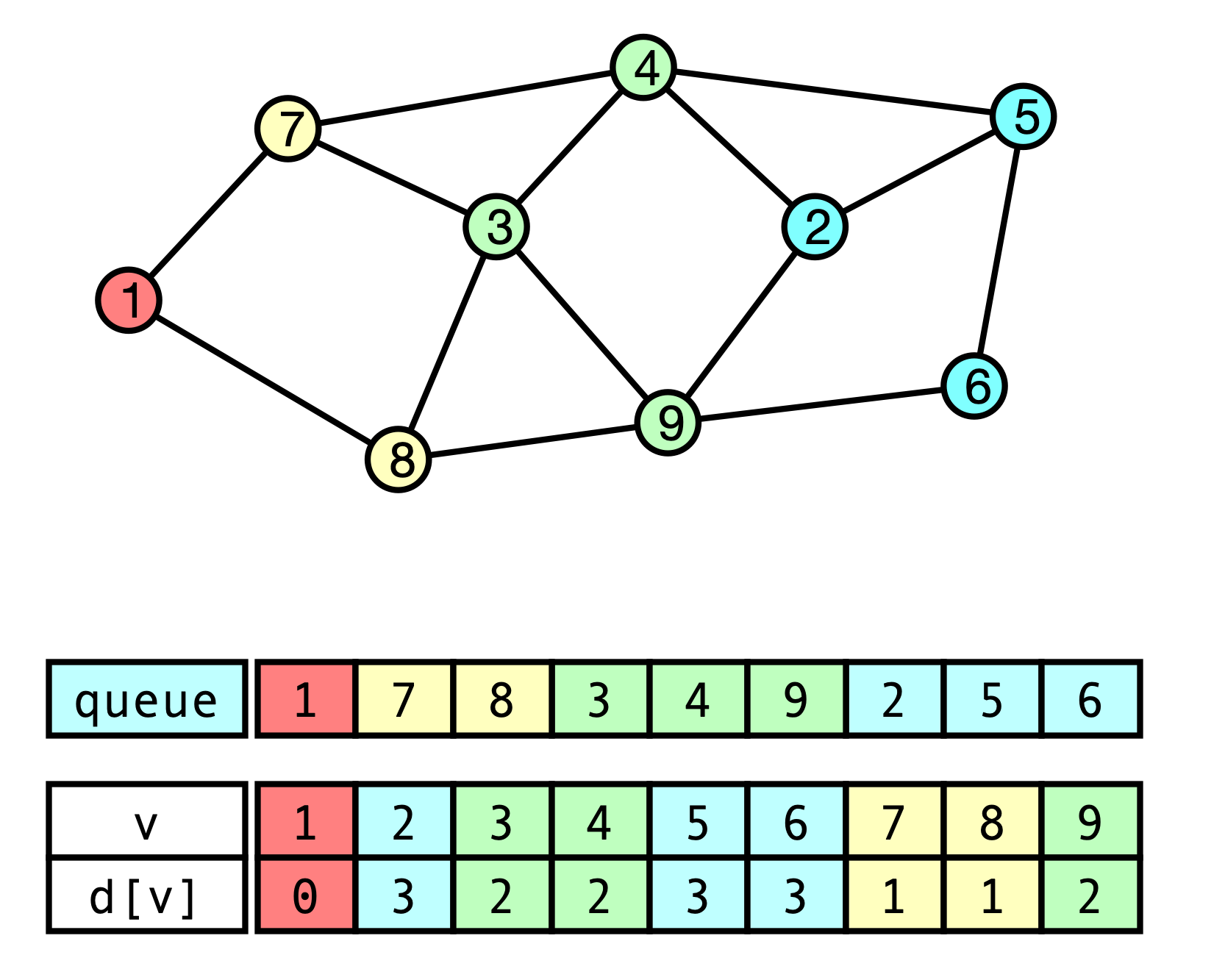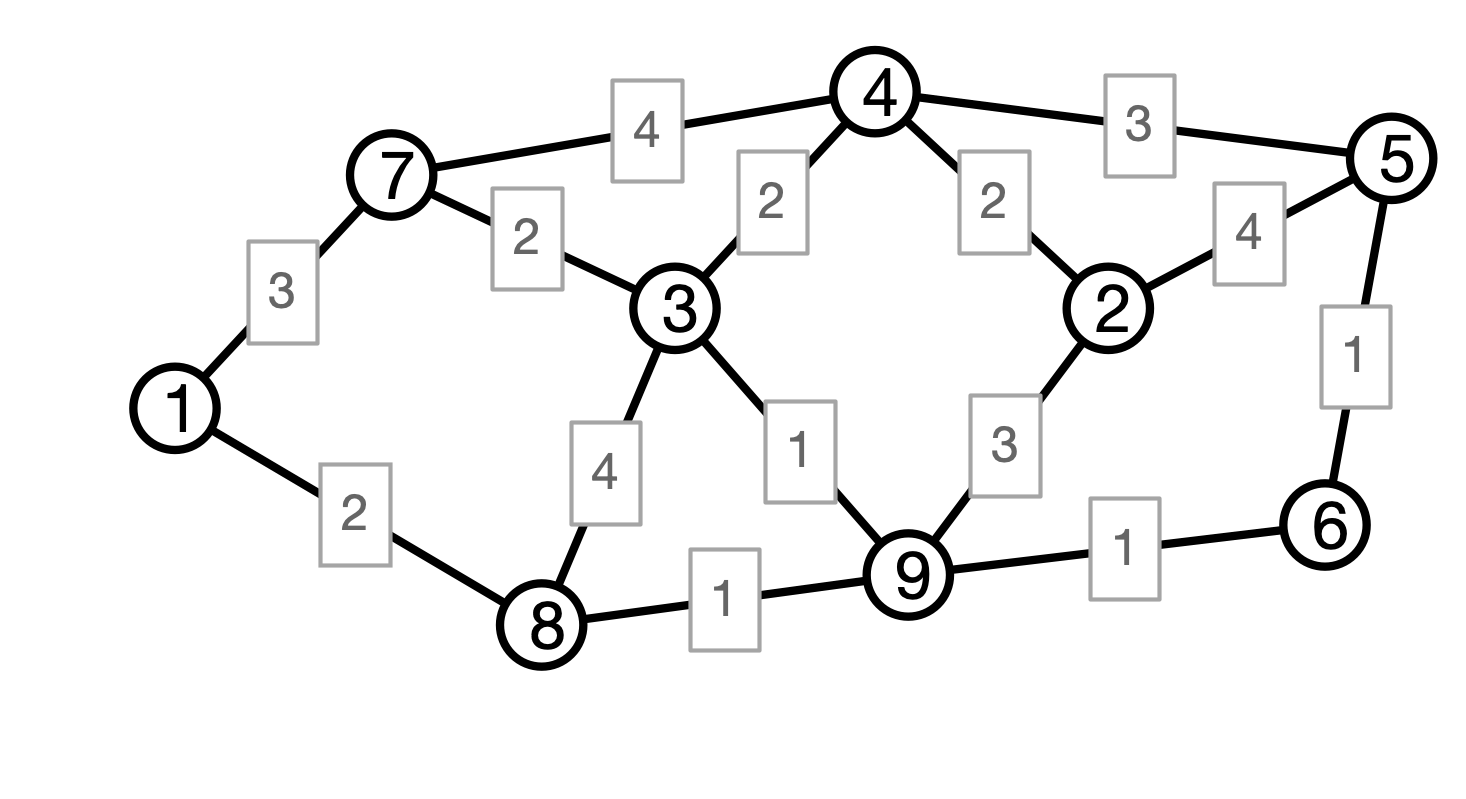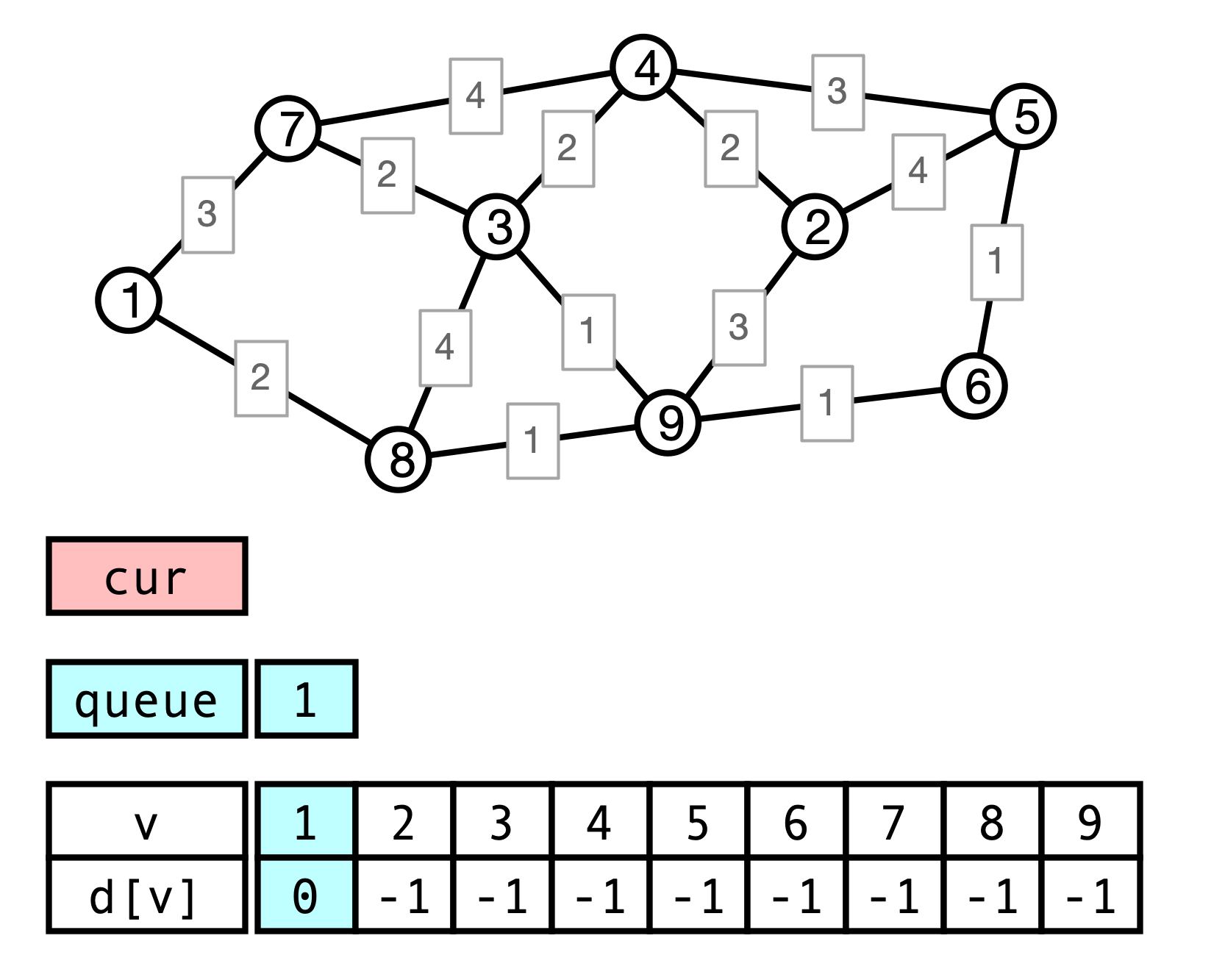# Lecture 15: Graphs and Distances

### COSC 311 Algorithms, Fall 2022

$\def\compare{ {\mathrm{compare}} } \def\swap{ {\mathrm{swap}} } \def\sort{ {\mathrm{sort}} } \def\insert{ {\mathrm{insert}} } \def\true{ {\mathrm{true}} } \def\false{ {\mathrm{false}} } \def\BubbleSort{ {\mathrm{BubbleSort}} } \def\SelectionSort{ {\mathrm{SelectionSort}} } \def\Merge{ {\mathrm{Merge}} } \def\MergeSort{ {\mathrm{MergeSort}} } \def\QuickSort{ {\mathrm{QuickSort}} } \def\Split{ {\mathrm{Split}} } \def\Multiply{ {\mathrm{Multiply}} } \def\Add{ {\mathrm{Add}} } \def\cur{ {\mathrm{cur}} }$

## Overview

1. Single Source Shortest Paths
2. Depth First Search
3. Weighted Graphs
4. Weighted Shortest Paths

## More Bridges

Gephyrophobia = fear of bridges

Question. How to get from one landmass to another, crossing the fewest possible number of bridges?

## Strategy

Find shortest (fewest hops) route by:

1. find all vertices reachable in 1 hop
2. find all vertices reachable in 2 hops
3. find all vertices reachable in 3 hops

Continue until destination is found

## Graph Distances

Definition. $G = (V, E)$ a graph, $u, v \in V$ vertices. The graph distance between $u$ and $v$, denoted $d(u, v)$, is the length of the shortest path from $u$ to $v$ in $G$.

## ExampleWhat is $d(1, 3)$? What is $d(1, 5)$?

## Single Source Shortest Paths (SSSP)

Unweighted version

Input.

• a Graph $G = (V, E)$
• an initial vertex $u \in V$
• each vertex $v \in V$ has associated adjacency list
• list of $v$’s neighbors

Output.

• A map $d_u: V \to \{-1, 0, 1, 2, \ldots\}$ such that $d_u(v) = d(u, v)$ is the graph distance from $u$ to $v$

• $d[v] = -1$ indicates no path from $u$ to $v$

## Example## BFS Solution

1. start at $u$
2. examine $u$’s neighbors, at distance $1$
3. examine $u$’s neighbors’ neighbors, at distance $2$
4. $\vdots$

Greedily examine closest vertices that have not yet been examined…

## Queues

• stores elements
• two basic operations
• add(x) adds element x to queue
• remove() removes and returns element
• FIFO: first in, first out

## BFS Pseudocode

  BFS(V, E, u):
intialize d[v] <- -1 for all v
d[u] <- 0
while queue is not empty do
v <- queue.remove()
for each neighbor w of v do
if d[w] = -1 then
d[w] <- d[v] + 1
return d


## BFS Correctness

Theorem. When BFS(V, E, u) terminates, for every vertex $v \in V$, $d[v]$ stores the distance (minimum number of hops) from $u$ to $v$.

Analysis. Break $V$ into layers

• $L_0$ contains only $u$
• $L_1$ contains neighbors of $u$
• $L_2$ contains neighbors of neighbors of $u$
• $\vdots$
• $L_k$ contains vertices not in $L_0, \ldots, L_{k-1}$ but with at least one neighbor in $L_{k-1}$

## Layered Illustration## More Formally

For $i = 0, 1, 2, \ldots$, Define $L_i$ by

• $L_0 = \{u\}$
• $L_i =$ vertices not in $L_0, L_1, \ldots, L_{i-1}$ that have at least one neighbor in $L_{i-1}$

Claim. $L_i$ contains precisely the vertices in $V$ at distance $i$ from $u$.

## Analysis of BFS

To Show

1. procedure finds vertices in increasing order of distance
2. distances are correctly computed when vertex is found (added to queue)

Idea. Break execution of BFS into phases

• phase $i$ starts when first element of $L_i$ is added to queue
• phase $i$ ends when last element in $L_i$ is added to queue

## Phase Illustration## Phase Claim

Claim. Consider an execution of BFS procedure. Then for every phase $i$:

1. phase $i$ ends before phase $i+1$ begins
2. every vertex from $L_i$ is added to the queue in phase $i$
3. each vertex $v$ added in phase $i$ has $d[v] = i$

Proof. Use induction on $i$

Base case $i = 0$. $u$ is the only element in $L_0$, and it is added before any other elements, and $d[u]$ is initialized to $0$.

## Inductive Step of Phase Claim

Suppose claim holds for $j \leq i$ (inductive hypothesis). Then:

• when phase $i$ ends (1) all vertices from $L_i$ are in queue and (2) no vertex in $L_{i+1}$ is in queue
• start removing elements in $L_i$ from queue
• when $v$ in $L_i$ is removed, any neighbors in $L_{i+1}$ are added to queue (if not already)
• distance is set to $d[v] \leftarrow i+1$
• every $v$ in $L_{i+1}$ has neighbor in $L_i$
• $\implies$ all $v \in L_{i+1}$ are added to queue when last $L_i$ vertex is removed from queue
• no vertex in $L_{i+2}$ added to queue to this point

## Conclusion

  BFS(V, E, u):
intialize d[v] <- -1 for all v
d[u] <- 0
while queue is not empty do
v <- queue.remove()
for each neighbor w of v do
if d[w] = -1 then
d[w] <- d[v] + 1
return d


BFS procedure correctly computes all distances from $u$!

## What is Running Time of BFS?

  BFS(V, E, u):
intialize d[v] <- -1 for all v
d[u] <- 0
while queue is not empty do
v <- queue.remove()
for each neighbor w of v do
if d[w] = -1 then
d[w] <- d[v] + 1
return d


## More General Problem

Definition. A weighted graph is a graph $G(V, E)$ where each edge $e \in E$ is additionally assigned a (real valued) weight $w(e)$.

• for now, assume $w(e) \geq 0$

Examples.

• weights = distances (not just number of hops)
• weights = cost of connection
• weights = latency of connection

## Distance in Weighted Graphs

• $G = (V, E)$ a graph, $w$ weights

• $P = v_0 e_1 v_1 e_2 v_2 \cdots e_k v_k$ a path

• The (weighted) length of $P$ is

$w(P) = w(e_1) + w(e_2) + \cdots + w(e_k)$

## Example## Weighted Shortest Paths

Given weights $w$, define $d_w(u, v)$ to be minimum (weighted) length of any path $P$ from $u$ to $v$.

## ExampleWhat is $d_w(1, 3)$? What about $d_w(1, 5)$?

## Weighted SSSP

Input.

• a weighted Graph $G = (V, E)$, edge weights $w$
• an initial vertex $u \in V$
• each vertex $v \in V$ has associated adjacency list
• list of $v$’s neighbors
• includes weight of edge from $v$ to each neighbor

Output.

• A map $d_u: V \to \mathbf{R}$ such that $d_u(v) = d(u, v)$ is the graph distance from $u$ to $v$

• $d[v] = \infty$ indicates no path from $u$ to $v$

## Weighted SSSP

Does BFS compute weighted distances from $u$?

• must update procedure
• when processing edge $(v, x)$, should updated $d[x] = d[v] + w(v, x)$ rather than setting $d[x] <- d[v] + 1$

Does this work?

## Weighted BFS Example## Issue

• BFS processes vertices in order of fewest hops from $u$
• With weighted graphs, shortest path need not have fewest hops

## BFS Analysis Takeaway

• BFS succeeds on unweighted graphs because closer vertices are processed before farther vertices

• Could we get similar behavior for weighted distances?

• must ensure: vertices processed in order of weighted distance from $u$

• how can we do this?

• How could we efficiently implement a modified procedure?

## Next Time

Dijkstra’s Algorithm